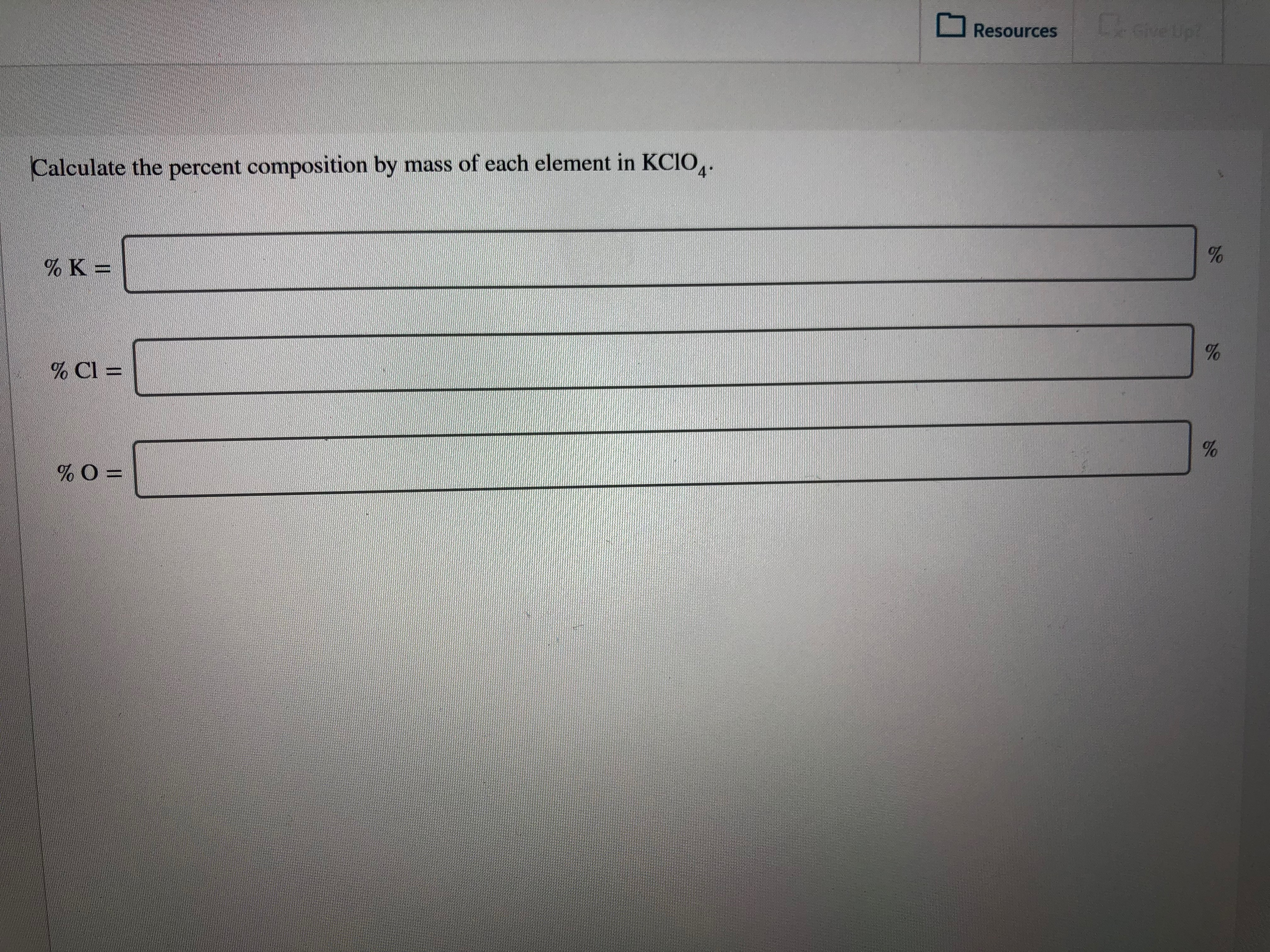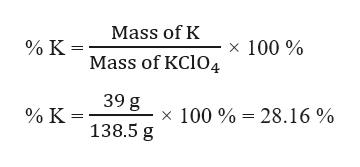# Lave UptResources4Calculate the percent composition by mass of each element in KCIO,.% K =%% Cl =% O =

Question
1 viewshelp_outlineImage TranscriptioncloseLave Upt Resources 4 Calculate the percent composition by mass of each element in KCIO,. % K = % % Cl = % O = fullscreen
check_circle

Step 1

The given compound is KClO4

Molar mass of K = 39 g/mol

Molar mass of Cl = 35.5 g/mol

Molar mass of O = 16 g/mol

Molar mass of KClO4 = 39 + 35.5 + (4×16) = 138.5 g/mol

Step 2

1 mole of KClO4 (138.5 g) contains 1 mole of K (39 g).

Therefore, the mass % of K can be calculated as:help_outlineImage TranscriptioncloseMass of K x 100% % K = Mass of KCIO4 39 g % K x 100% 28.16 % 138.5 g fullscreen
Step 3

1 mole of KClO4 (138.5 g) contains 1 mole of Cl (35.5 g).

There...

### Want to see the full answer?

See Solution

#### Want to see this answer and more?

Solutions are written by subject experts who are available 24/7. Questions are typically answered within 1 hour.*

See Solution
*Response times may vary by subject and question.
Tagged in

### General Chemistry